Year 9 Interactive Maths - Second Edition

## Triangles

Architects and builders use triangles as they provide greater rigidity to the structure of a building.  Most roofs have a triangular cross-section.  In this section, we will consider the main features of triangles and their use in solving numerical problems.

A triangle is a closed plane figure bounded by three line segments.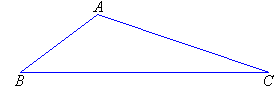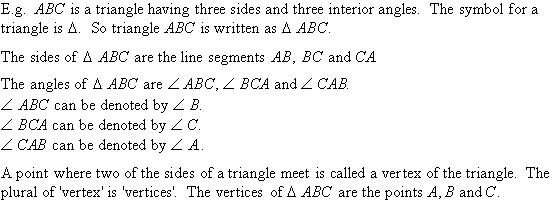###### Recall that:

The angle sum of a triangle is 180º.

## Types of Triangles

There are four basic types of triangles:

### Scalene triangles

A scalene triangle has no equal sides.### Isosceles triangles

An isosceles triangle has two sides equal.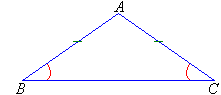The angles opposite the equal sides are equal.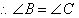### Equilateral triangles

An equilateral triangle has all sides equal.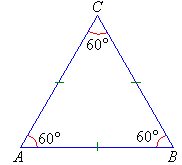All angles of an equilateral triangle are 60º.

### Right triangles

A right triangle has one angle of 90º, called a right angle.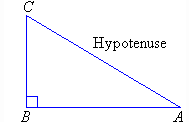The side opposite the right angle is called the hypotenuse.  The hypotenuse is the longest side of the triangle (use a ruler to verify this).

###### Note:

In the diagrams, equal lengths and equal angles are each marked in the same way.

#### Example 7

Find the values of the pronumerals x and y in the following diagram.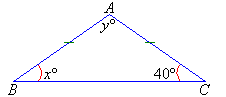##### Solution: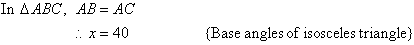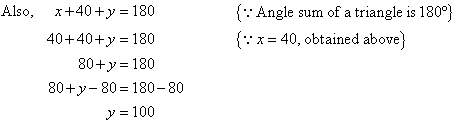So x = 40, y = 100.

#### Example 8

Find the values of the pronumerals x and y in the following diagram.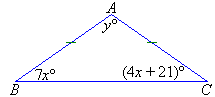##### Solution: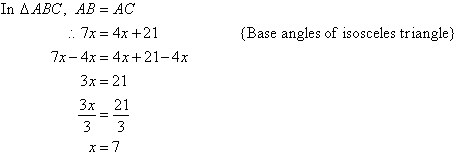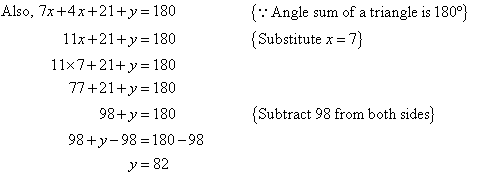So x = 7, y = 82.

#### Example 9

Find the value of the pronumeral x in the following diagram.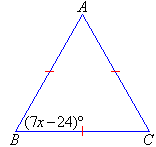##### Solution: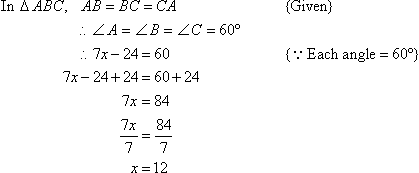So, x = 12.

#### Example 10

Find the values of the pronumerals x and y in the following diagram.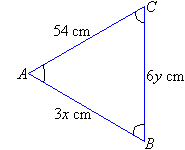##### Solution: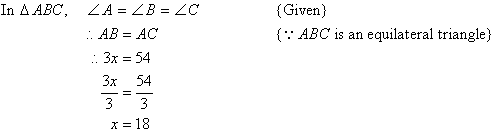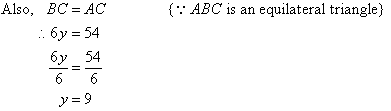So, x = 18, y = 9.

#### Example 11

Find the value of the pronumeral x in the following diagram.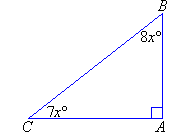##### Solution: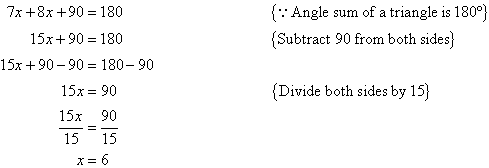So, x = 6.